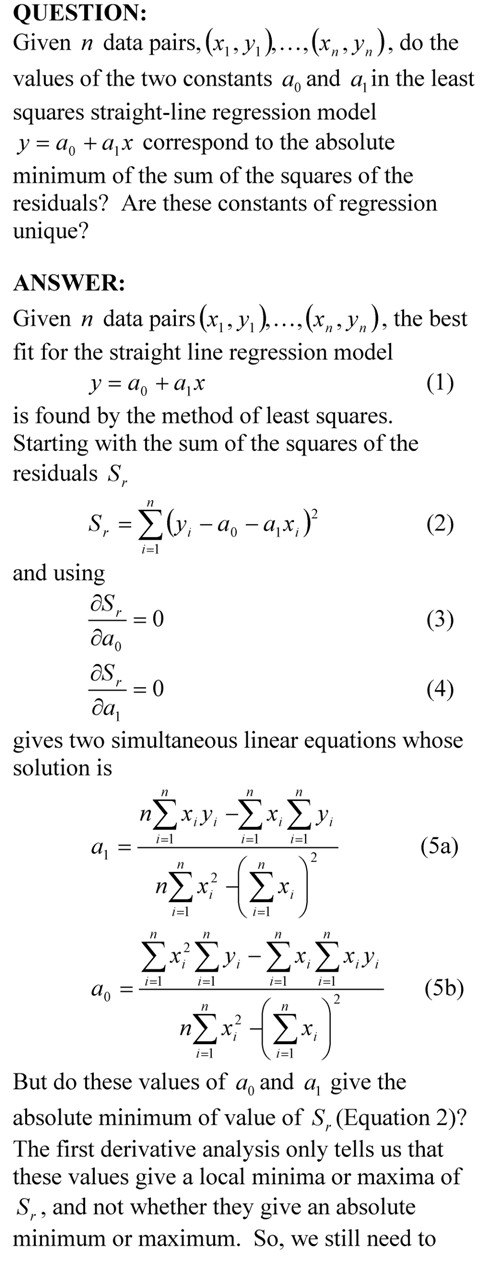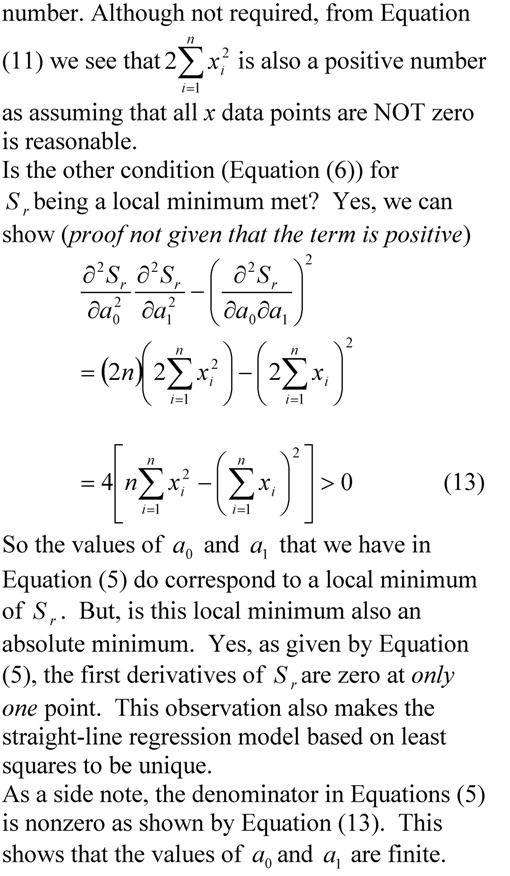## Prove that the least squares general straight-line model gives the absolute minimum of the sum of the squares of the residuals?

Many regression models when derived in books only show the first derivative test to find the formulas for the constants of a linear regression model.  Here we show a thoroughly explained derivation.___________________________________________

This post is brought to you by Holistic Numerical Methods: Numerical Methods for the STEM undergraduate at http://numericalmethods.eng.usf.edu, the textbook on Numerical Methods with Applications available from the lulu storefront, the textbook on Introduction to Programming Concepts Using MATLAB, and the YouTube video lectures available at http://numericalmethods.eng.usf.edu/videos.  Subscribe to the blog via a reader or email to stay updated with this blog. Let the information follow you.